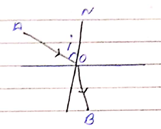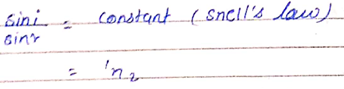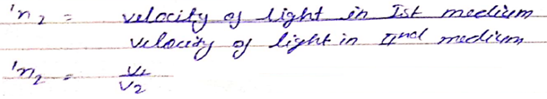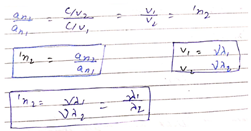# RAY OPTICS AND OPTICAL INSTRUMENT - Refraction of Light

The binding of the X-ray of light passing from one medium to the other medium is called Refraction.AO – Incident ray
OB – refracted ray
Angle i – Incident angle
Angle r – Refracted angle

Laws of Refraction: The ratio of the sign i of the angle of the incidence to the angle of refraction ( r) is a constant called refractive index.1n2 is the refractive index of second medium.If first medium is air, then velocity of light (v = c) and velocity of light is second medium (v2 = v)

1n2 = c / v (absolute refractive index)

Absolute refractive index 1st medium

an2 = c / v2

Refractive index of 2nd medium with respect to 1st mediumSecond Law: The incident ray, refracted ray and the normal to the interface at the point of interface, all lie in same plane.

Post By : Nek Singh Yadav 02 May, 2020 439 views Physics